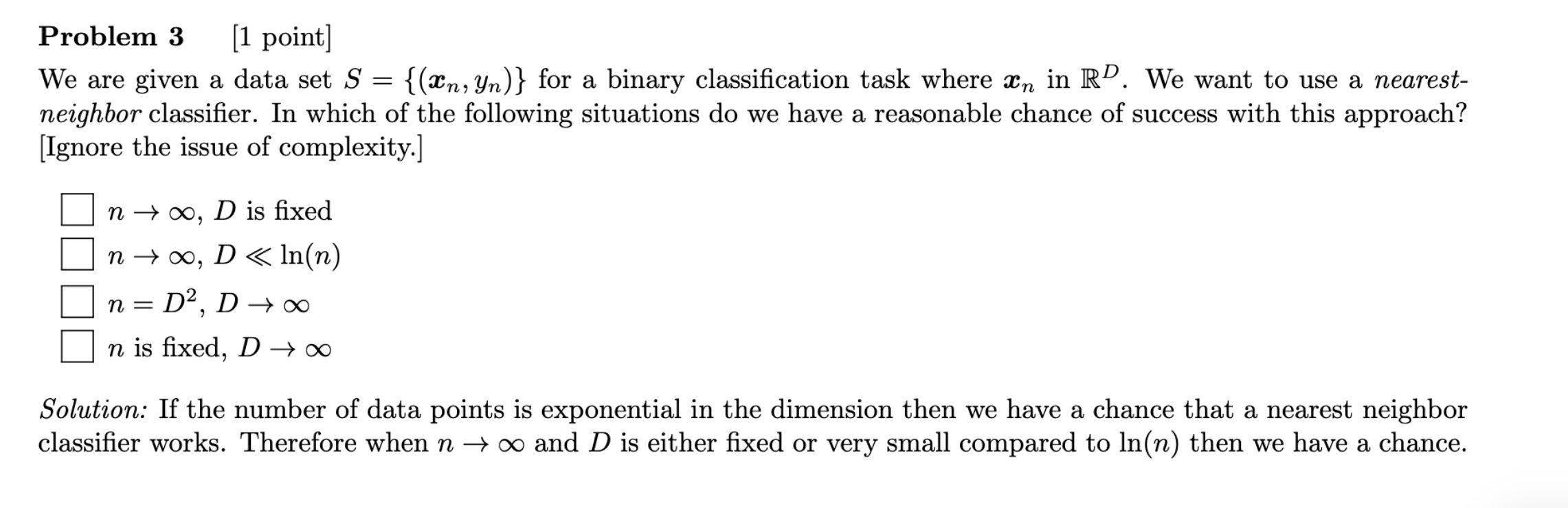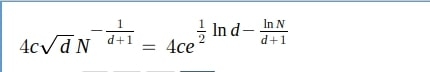### Q3 exam 2017

Hi,
How can we explain this Upper bound for the dimension "D" wrt to the size of the data set "n" in knn ? ( why is the 2nd answer true?)
Thanks in Advance!When we did KNN on lectures we mentioned this lemma:This lemma states that the loss of KNN classifier is bounded by twice as loss of Bayesian classifier (f*) plus some function that depends on N and D. We want that function to be as small as possible. It holds that:So, if ln N >> d, than -ln N/(d+1) tends to -infinity. If ln d -ln N/(d+1) also tends to -infinity, than the whole expression will tend to 0, and that is what we want. However, in order to assume that, I think that we need slightly stronger assumption: lnN>>d lnd.

Although it is not a complete answer, I hope this helps a bit

Page 1 of 1